Starts With A Bang

# Ask Ethan: Would a false vacuum state of the Universe lead to our destruction?

There's a big difference between the notions of 'false vacuum' and 'true vacuum' states. Here's why we don't want to live in the former.
Key Takeaways
• The vacuum is defined as the zero-point energy of empty space: how much energy-per-volume is left over after all physical quanta are removed.

• This value could have been zero, but isn't: it has a positive, non-zero value.

• If we live in a false, rather than true, vacuum, the vacuum could decay, with catastrophic consequences for the Universe.

One of the great existential worries that plagues the minds of theoretical physicists is that the vacuum of space might not be in its true vacuum state, but could instead reside in a false vacuum instead. If you were to remove everything you could imagine from a large region of space, including:

• matter,
• neutrinos,
• external electric and magnetic fields,
• and any gravitational sources or spacetime curvature,

you would be left with purely empty space, or as close as we can come to a physical definition of “nothing.” You might expect that if you were to draw an imaginary box around this region of “nothing” and measured the total amount of energy inside, you would find that it was precisely zero. But that’s not what we find; we find that there actually is a positive, non-zero amount of energy inherent to space itself, even if we remove all the identifiable quantum and classical sources of matter and energy. What does this mean for the nature of the quantum vacuum, and in particular for the distinction between “true vacuum” and “false vacuum?” That’s what Eric Mars wants to know, asking:

“Could you please explain what false vacuum and true vacuum mean and its implications in the existence of the universe.”

It’s a great question, and it requires that we start with the idea — specifically for physics — of zero.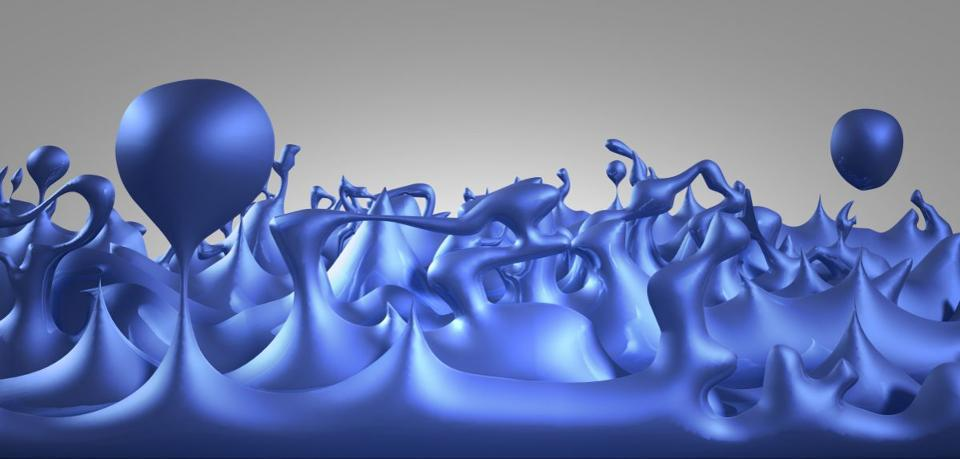This artist’s illustration depicts how the foamy structure of spacetime may appear, showing tiny bubbles quadrillions of times smaller than the nucleus of an atom that are constantly fluctuating and lasting for only infinitesimal fractions of a second. Rather than being smooth, continuous, and uniform, at the quantum scale, spacetime has fluctuations inherent to it, which likely correspond to a non-zero zero-point energy. (Credit: NASA / CXC / M. Weiss)

In mathematics, zero is simply a number, signifying the absence of either a positive or negative amount of any quantity. In physics, however, there is another way to define zero: the zero-point energy of a system, or the lowest possible energy state that it can achieve while still remaining the same system we were initially talking about. For any physical system we can dream up, there will be at least one configuration for that system that has the lowest total amount of energy in it. For any physical system you can imagine, there is always at least one lowest-energy configuration.

• If you have a collection of masses isolated from the remainder of the Universe, the lowest-energy configuration is a black hole.
• For a proton and an electron, the lowest-energy configuration is a hydrogen atom in the ground (n=1) state.
• And for the Universe itself, it is to have perfectly empty space in the absence of any internal or external fields or sources.

That lowest-energy configuration is known as the zero-point energy of a system. It would make sense — and for many of us, we would simply intuit that it’s so — if the zero-point energy of any system were defined as zero. But that is not quite how it works.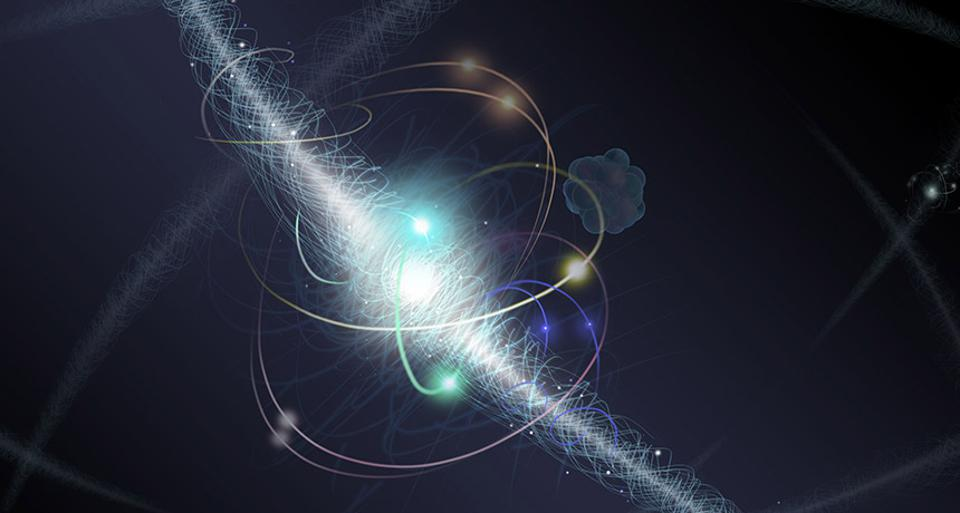This artist’s illustration shows an electron orbiting an atomic nucleus, where the electron is a fundamental particle, but the nucleus can be broken up into still smaller, more fundamental constituents. The simplest atom of all, hydrogen, is an electron and a proton bound together. But the lowest-energy configuration imaginable, where the electron just sits stationary at the center of the proton, never occurs. (Credit: Nicole Rager Fuller / NSF)

Take the hydrogen atom, for example: a single electron orbiting a single proton. If you think classically, you would imagine that the electron could orbit that proton at any radius at all, from a large one down to a small one. Just as a planet can orbit a star at any distance, based on their mutual masses and relative speeds, you would think that a negatively charged electron could orbit a positively charged proton at any distance as well, based simply on the speed of the orbit and the balance of kinetic and potential energy.

But this ignores an extraordinarily important property of nature: the fact that the Universe is fundamentally quantum mechanical, and that the only allowable energy levels for an electron orbiting a proton are quantized. As a result, there is a lowest possible energy state that a physical system such as this can have, and that does not correspond to the electron sitting at rest directly atop the proton (that is, the lowest imaginable energy state). Instead, there is a lowest-energy state that is physically allowable, which corresponds to the electron orbiting the proton in the n=1 energy state.

Even if you cool your system down to absolute zero, there will still be this finite, non-zero energy that your system will have.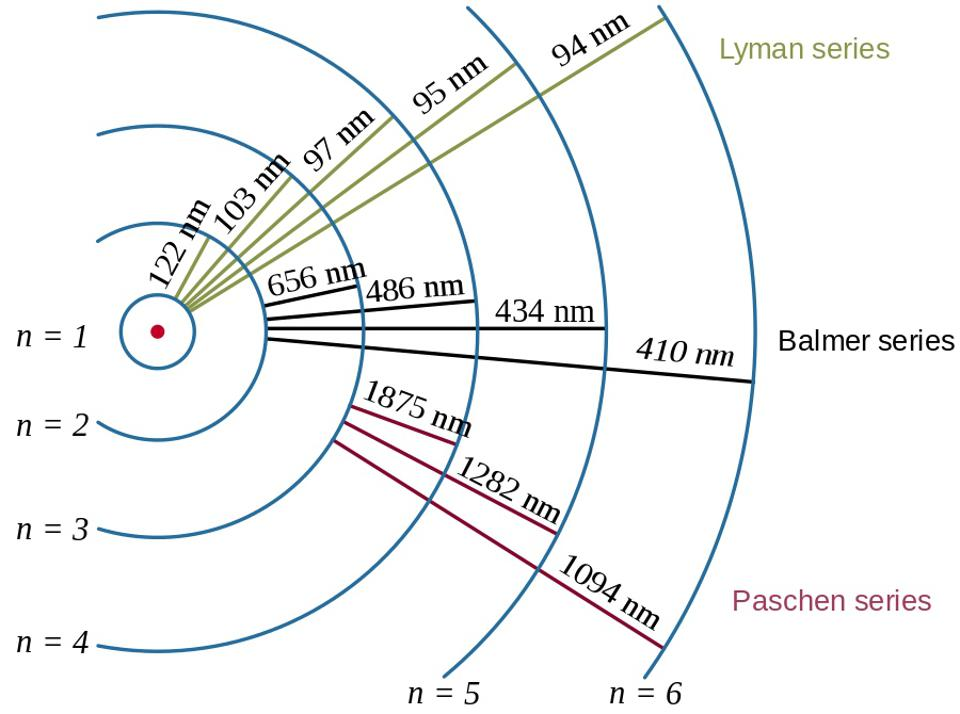Electron transitions in the hydrogen atom, along with the wavelengths of the resultant photons, showcase the effect of binding energy and the relationship between the electron and the proton in quantum physics. Hydrogen’s lowest energy state corresponds to the n=1 state: a ground state with a finite, positive, non-zero amount of energy. (Credit: OrangeDog and Szdori / Wikimedia Commons)

This idea, of a zero-point energy to any quantum mechanical system, goes all the way back to Max Planck in 1911 and was extended to fields by Einstein and his collaborator, Otto Stern (the same Stern who formulated the infamous Stern-Gerlach experiment), and a paper they wrote back in 1913. If we fast-forward to today, more than 100 years later, we now understand that our Universe is governed by a combination of General Relativity, our law of gravitation, and quantum field theory, which describes the other three fundamental forces.

The idea of a zero-point energy to the fabric of space itself shows up in both General Relativity and quantum field theory, but it comes about in vastly different ways. In General Relativity, the curvature of space is what determines the future motion of matter and energy through the Universe, while the presence and distribution and motion of matter and energy in turn determines the curvature of space. Matter and energy tell spacetime how to curve, and that curved spacetime tells matter and energy how to move.

Almost.

Why is this only “almost” true? Because, as anyone who has ever performed an indefinite integral (from calculus) will recall, you are free to add a constant to your answer: the dreaded “plus c.”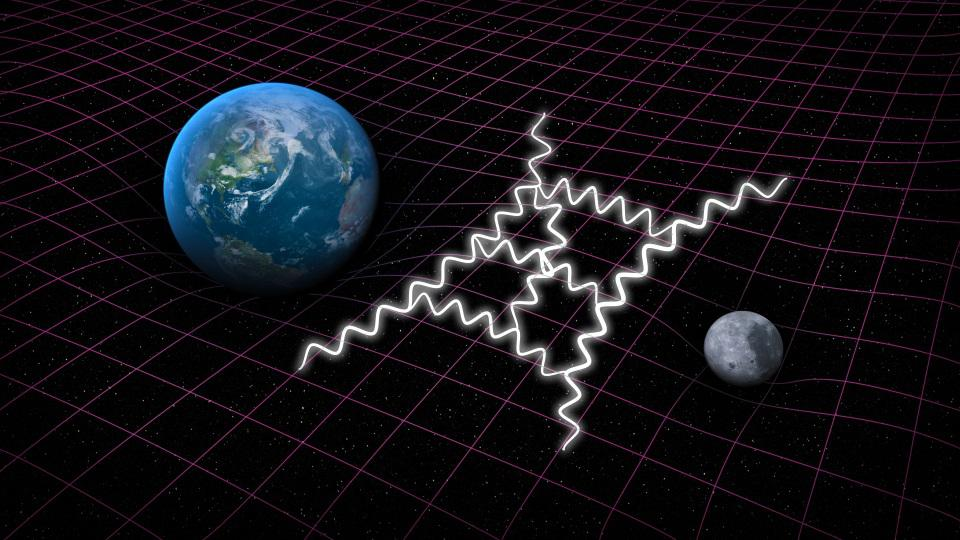In General Relativity, the presence of matter and energy determine the curvature of space. In quantum gravity, there will be quantum field theoretical contributions that lead to the same net effect. In addition to curved space, you can add a constant: a cosmological constant in General Relativity, which corresponds to the sum of all loop diagrams for the vacuum in quantum field theory. It is possible that the quantum gravity contributions to the zero-point energy of space are responsible for the dark energy we see in our Universe today, but that is just one of many viable possibilities. (Credit: SLAC National Accelerator Laboratory)

In General Relativity, this constant comes into play as a cosmological constant, and it can take on any positive or negative value that we like. When Einstein wanted to construct a static Universe, he threw in a positive constant to keep his toy model of the Universe — one where masses were evenly distributed infinitely throughout space — from collapsing; the cosmological constant would counteract gravitational attraction. There was no reason for this constant to have the positive, non-zero value that he assigned to it. He simply asserted it must be so, otherwise the Universe could not be static. With the discovery of the expanding Universe, the constant was no longer needed, and was discarded for more than 60 years.

On the other hand, there is quantum field theory, too. Quantum field theory encourages you to imagine all the ways that particles can interact with one another, including via the creation/annihilation of particle-antiparticle pairs as intermediate steps, radiative corrections, and any other sets of interactions that arn’t forbidden by the laws of quantum physics. It then goes a step farther, however, which most people may not recognize. It says that in addition to these interacting fields in the presence of matter and energy, there are “vacuum” contributions, which represent how quantum fields in the vacuum of space, with no particles present at all, behave.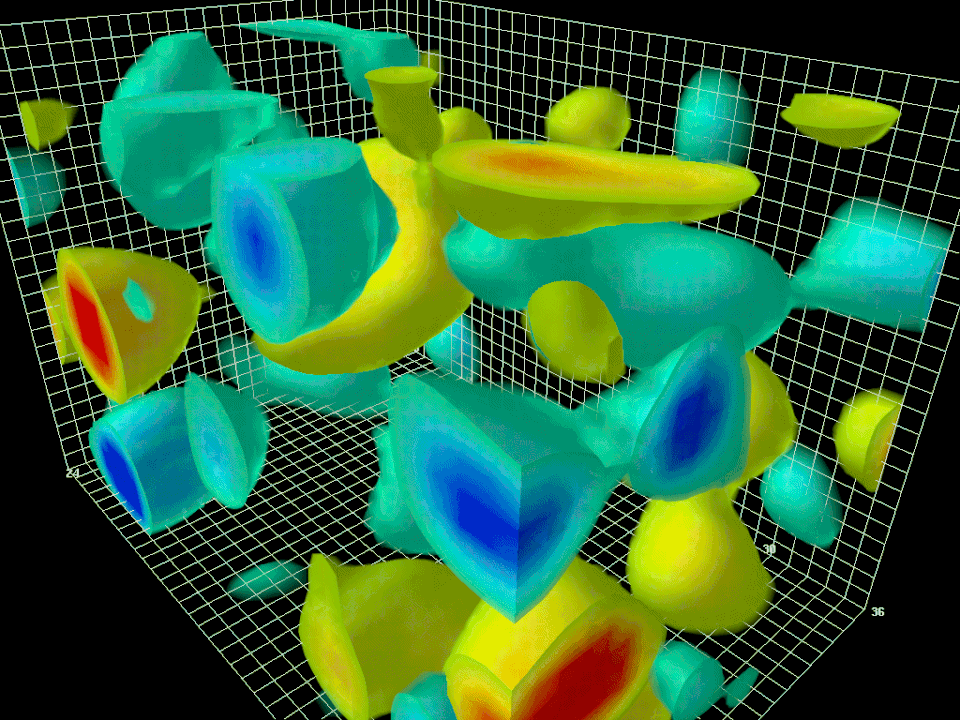Visualization of a quantum field theory calculation showing virtual particles in the quantum vacuum (specifically, for the strong interactions). Even in empty space, this vacuum energy is non-zero, and what appears to be the “ground state” in one region of curved space will look different from the perspective of an observer where the spatial curvature differs. As long as quantum fields are present, this vacuum energy (or a cosmological constant) must be present, too. (Credit: Derek Leinweber)

Now, here’s where things get uncomfortable: we do not know how to calculate the zero-point energy of space from these quantum field theory methods, either. Each individual channel that we know how to calculate can contribute to this zero-point energy, and the way we find an individual contribution is to calculate what we call its vacuum expectation value. The problem is each such channel has an enormous vacuum expectation value: more than 100 orders of magnitude too large to be possible. Some channels have positive contributions and others have negative contributions.

Being unable to make a sensible calculation, we made an ignorant assumption: that all of the contributions would cancel out, summing to zero, and that the zero-point energy of space would, in fact, be precisely equal to zero.

Then, in the 1990s, something changed again. Observations of the Universe began to indicate that there was something causing the Universe’s expansion to accelerate, and that “thing,” whatever it is, was consistent not with any form of matter or radiation, but rather with a positive, non-zero amount of zero-point energy to the fabric of space itself. We had just measured the value of the vacuum energy inherent to space, and it was very small, but very importantly, greater than zero.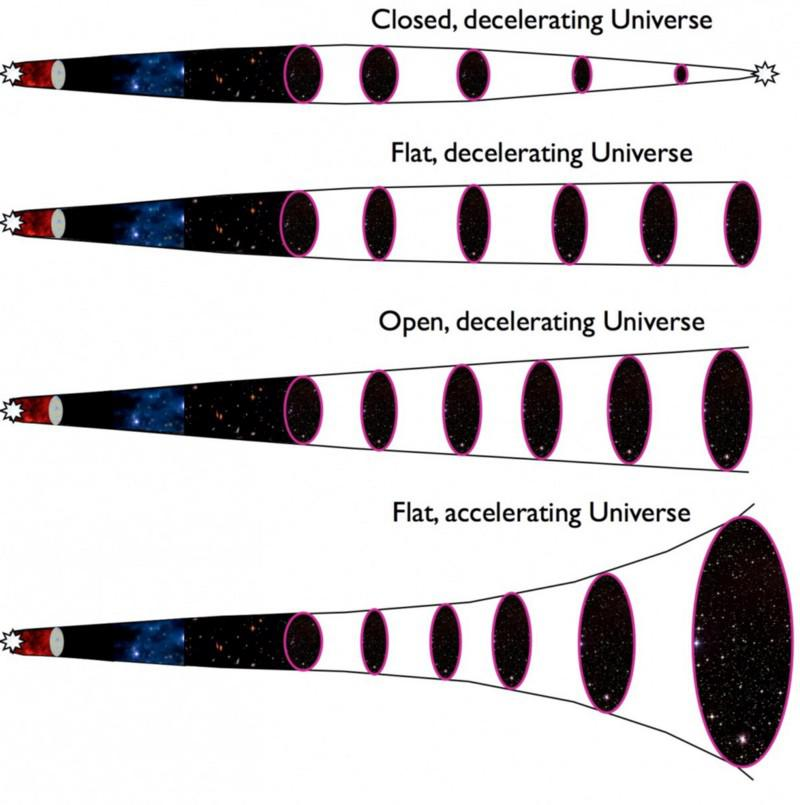The expected fates of the Universe (top three illustrations) all correspond to a Universe where the matter and energy combined fight against the initial expansion rate. In our observed Universe, a cosmic acceleration is caused by some type of dark energy, which is hitherto unexplained. All of these Universes are governed by the Friedmann equations, which relate the expansion of the Universe to the various types of matter and energy present within it. (Credit: E. Siegel / Beyond the Galaxy)

This opened up a slew of questions.

• Was this form of energy — what we now call dark energy — exactly a cosmological constant or not? (The answer is yes, at least, to the precision that we can measure it.)
• Did it remain constant throughout time, or did it strengthen or weaken? (The answer: it’s consistent with being a perfect constant.)
• Could we ever hope to calculate it based on what we know about quantum field theory? (The answer: we don’t know, but we are arguably no closer today than we were 20+ years ago.)
• And, worryingly, is the zero-point energy we are observing the true vacuum of space, or is it merely a false vacuum? (We don’t know.)

Why would we worry about the last one? Because the most important property of the vacuum of space isn’t what the precise value of the zero-point energy is; rather, it’s vital to our Universe’s stability that the vacuum of space has a zero-point energy that doesn’t change. And just as a hydrogen atom in any excited state will have the capability of transitioning to a lower-energy state on its way down to the zero-point state, a Universe in a false vacuum will remain capable of transitioning to a true vacuum (or a lower-energy but still false vacuum) state.If you draw out any potential, it will have a profile where at least one point corresponds to the lowest-energy, or “true vacuum,” state. If there is a false minimum at any point, that can be considered a false vacuum, and it will always be possible, assuming this is a quantum field, to quantum tunnel from the false vacuum to the true vacuum state. (Credit: Stannered / Wikimedia Commons)

You can think of this the same way you would think about starting a ball atop a mountain and allowing it to roll down — and down, and down, and down some more — until it finally came to rest. If your mountainside is smooth, you can imagine that you would easily roll all the way down into the lowest part of the valley beneath the mountain, where it would settle. That’s a true vacuum state: the lowest-energy state there is, where it’s not physically possible to transition to a lower-energy state. In a true vacuum, you’re already as low as you can go.

But if your mountainside is craggy, with pits, divots, moguls, and glacial lakes, you can imagine that your ball might come to rest somewhere other than the lowest possible point. Any other place it can remain for an indefinite period of time is not the true minimum but rather a false one. If we are talking about the vacuum state of the Universe, that means anything other than the lowest possible state is a false vacuum state.

Given that we have a positive, nonzero value for the cosmological constant in our Universe, it’s certainly possible that we live in a false vacuum state, and that the true vacuum, whatever it may be, exists at some other, lower-energy state.This generic illustration of quantum tunneling assumes there is a high, thin, but finite barrier separating a quantum wavefunction on one side of the x-axis from the other. While most of the wavefunction, and hence the probability of the field/particle that it’s a proxy for, reflects and remains on the original side, there is a finite, non-zero probability of tunneling through to the other side of the barrier. (Credit: Yuvalr / Wikimedia Commons)

Now, it might also not be the case; we may be in the true vacuum state. If so, there is no possibility of transitioning to a lower-energy state, and here we will remain for the remainder of our Universe’s existence.

But what if we live in a false vacuum state? Well, in a quantum Universe, no matter how large the distance is between a false and true minimum, how high the barrier is separating the false and true minimum, or how quickly or slowly the quantum mechanical wavefunction describing your state spreads out, there is always a finite, greater-than-zero probability of quantum tunneling from the higher-energy to the lower-energy state.

This is usually referred to as the vacuum catastrophe, because if we do quantum tunnel to a lower energy state, we have no reason to believe that the laws and/or constants that govern the Universe will remain unchanged. Wherever this vacuum decay occurs, things like atoms, planets, stars, and yes, human beings, will all be destroyed. This “bubble of destruction” will propagate outward at the speed of light, which means if it occurs, right now, anywhere within about 18 billion light-years of us, we will eventually be destroyed by it. This may even be suggested by our best measurements of the properties of the fundamental particles, which indicates that the electroweak force, one of the fundamental forces of nature, may be inherently metastable.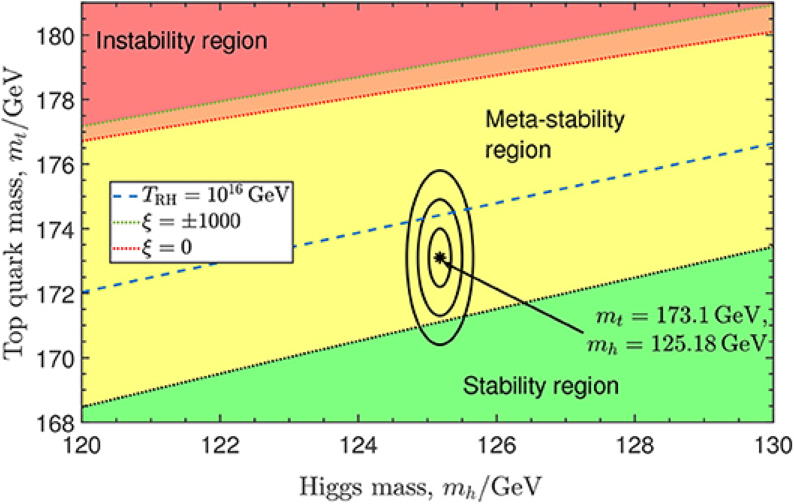Based on the masses of the top quark and the Higgs boson, we could either live in a region where the quantum vacuum is stable (true vacuum), metastable (false vacuum), or unstable (where it cannot stably remain). The evidence suggests, but does not prove, that we are in a region of false vacuum. (Credit: T. Markkanen, A. Rajantie and S. Stopyra, Front. Astron. Space. Sci., 2018)

It’s a grim thought, especially because we would never see it coming. One day, we would simply awaken to this wave of destruction that comes upon us at the speed of light, and then we would all be gone. In some ways, it is the most painless way to go that we can imagine, but it is also one of the saddest. Our cosmic legacy — of all that ever was, is, or will be — would instantaneously come to an end. All of the work that 13.8 billion years of cosmic evolution has done to create a Universe teeming with the ingredients for life, and possibly countless realizations of it, would be forever wiped out.

And yet, it is possible that something similar to this has already occurred: with the end of cosmic inflation and the onset of the hot Big Bang. A transition from a presumably very, very high energy vacuum state to a much lower-energy one, albeit a fundamentally different type of transition from quantum tunneling, is what brought inflation to an end and filled our Universe with matter and radiation some 13.8 billion years ago. Nevertheless, the possibility that we live in a false vacuum should remind us of how fleeting and fragile, and dependent upon the stability of the laws of physics, everything in our Universe is. If we live in a false vacuum state, and we could, every moment of existence could be our last.

Send in your Ask Ethan questions to startswithabang at gmail dot com!

Related

Up Next